Engineering Math - Quick Reference                                 Home : www.sharetechnote.com

SVD (Singular Value Decomposition)

Singluar Value Decomposition is a technique which decompose a matrix into three components as follows. The diagonal indicate the eigen values and the Unitary Matrix parts indicates the basis of each components. Depending on the nature of the original matrix M, you can interprete the U, V, S in many different ways. (Check Unitary Matrix page if you are not familiar with the concept and property of this matrix)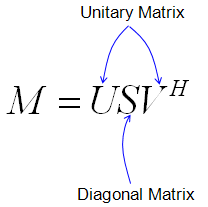If we do some dimension analysis and look into the meaning of each component matrix, it can be illustrated as follows.

(Look into Orthonormal matrix and Eigenvector page if you are not familiar with the concept and property of those matrix)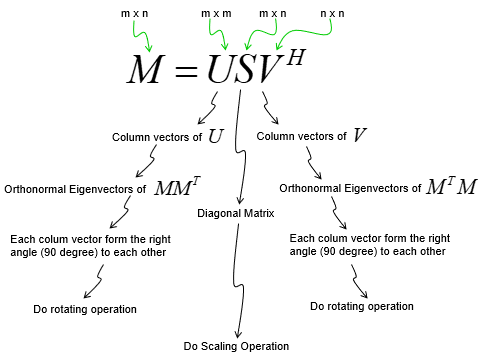This technique has various applications like PCA (Principal Component Analysis) in Statistics and MIMO channel analysis in radio communications and solving a linear system equation (linear Matrix equation) etc. (I put the link for some of the YouTube video showing the applications of SVD in Reference Section).

With this, we can decompose a matrix into three matrices that perform following three transformation in sequence. (SVD is tightly related to PCA (Principal Component Analysis))

• a first rotation in the input space
• a simple positive scaling that takes a vector in the input space to the output space
• another rotation in the output space

If you look into each matrix and meaning of matrix elements, it can be illustrated as follows. Each u vectors are orthogonal to each other and each v vectors are orthogonal to each other.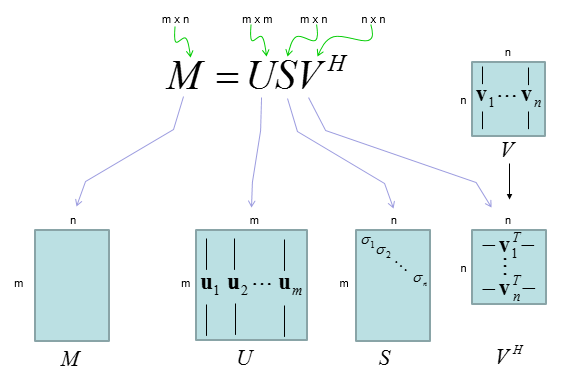NOTE :

• u1,u2,..,um is orthogonal to each other. It means if you draw the vector in cartesian coordinate they form right angle (90 degree) to each other.
• v1,v2,..,vn is orthogonal to each other. It means if you draw the vector in cartesian coordinate they form right angle (90 degree) to each other.

Geometrical Interpretation of SVD

I will try to represent the properties (each steps) of SVD in geometric form to help you to get some intuitive understanding. But understanding the meaning of the graphical representation itself may not always be easy and sometimes would confuse you even further. Just try to look into each of the plots and try to correlate the graph to the mathematical representation shown above. I hope there can be a way to get you understand this concept just by looking at something.. but unfortunately there is no such a thing in learning anything. You just get small pieces of help from here and there and gradually build up your own intuition. I hope this section can give you at least some help rather than total confusion :)

As you recall from the early part of this page, a matrix can magnify or rotate or shear the set of dots(points in a coordinate). SVD is a method to convert the whole transformational process into each separate transformation component, that is, rotational component, magnifying component and another rotational component. A graphical presentation of SVD is as follows. (I also posted the Octave/Matlab script that I wrote for this illustration and play with various other matrix('tm'  in the script) and see the result until get some intuitive feeling about this process).

There are many different ways to visualize SVD operation. Following is the way I visualize the SVD operation. The intention to this illustration is to show how each componet of SVD transform the orignal data(input). It may not make sense to you right away, but give you some time to think of each of the plot with the description below, it will (hopefully) gradually make sense.

(1) : this is the set of input vectors (each red dot indicate a vector) that will be transformed by the matrix M

(2) : this shows the result of the transformation performed by the matrix M

(3) : this shows the transformation done by U component of SVD. Here, you see that U perform rotation operation only. It does not do any scaling. It does not do any shearing.

(4) : this shows the transformation done by S component of SVD. Here, you see that S perform scaling operation only. It does not do any rotation. It does not do any shearing

(5) : this shows the transformation done by V^H( or transpose(V)) component of SVD. Here, you see that V^H perform rotation operation only. It does not do any scaling. It does not do any shearing

(8) : Same as (5)

(7) : Shows the transformation that step (5) and step (4) do together

(8) : Shows the transformation that step (5), step (4) and step (3) do together. This is same as the transformation that M matrix does.I put some more examples with matlab code for practice. try to interpret each of the plots yourself.

Look at the graphs on the top row. The red dots on left graph are transformed by the matrix M to be the blue dots on right graph.

Look at the graph on the middle row. The dots on left graph is the result of transforming M with the matrix U. You can see here that the area of the original rectangle(array of red dots) does not changes, but it get rotated. The dots on  the graph in the middle is the result of transforming M with the matrix S. You don't see any rotation here, but you see the original rectangle (array of red dots) get extended in x direction and contracted in y direction. The dots on right graph is the result of transforming M with the matrix V*(V Hermitian). You can see here that the area of the original rectangle(array of red dots) does not changes, but it get rotated.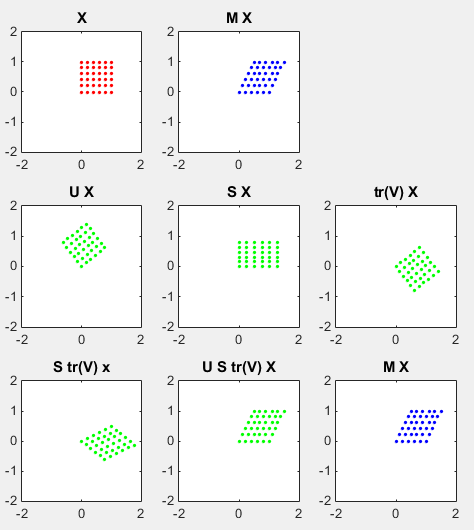clear all;

ptList_x=[];

ptList_y=[];

for y=0.0:0.2:1.0

for x=0.0:0.2:1.0

ptList_x=[ptList_x x];

ptList_y=[ptList_y y];

end

end

ptList_v = [ptList_x' ptList_y'];

ptList_v = ptList_v';

%tm = [1.25 1;0.2 0.9]

%tm = [1.0 0.5;0.0 1.0]

%tm = [1.2 0.0;0.0 1.5]

%tm = [cos(pi/6) -sin(pi/6);sin(pi/6) cos(pi/6)]

tm = [1.0 0.5;0.0 1.0]

[U,S,V]=svd(tm)

ptList_v_tm = tm * ptList_v;

ptList_v_tm_x = ptList_v_tm(1,:);

ptList_v_tm_x = ptList_v_tm_x';

ptList_v_tm_y = ptList_v_tm(2,:);

ptList_v_tm_y = ptList_v_tm_y';

ptList_v_tm_u = U * ptList_v;

ptList_v_tm_u_x = ptList_v_tm_u(1,:);

ptList_v_tm_u_x = ptList_v_tm_u_x';

ptList_v_tm_u_y = ptList_v_tm_u(2,:);

ptList_v_tm_u_y = ptList_v_tm_u_y';

ptList_v_tm_s = S * ptList_v;

ptList_v_tm_s_x = ptList_v_tm_s(1,:);

ptList_v_tm_s_x = ptList_v_tm_s_x';

ptList_v_tm_s_y = ptList_v_tm_s(2,:);

ptList_v_tm_s_y = ptList_v_tm_s_y';

ptList_v_tm_v = (conj(V)') * ptList_v;

ptList_v_tm_v_x = ptList_v_tm_v(1,:);

ptList_v_tm_v_x = ptList_v_tm_v_x';

ptList_v_tm_v_y = ptList_v_tm_v(2,:);

ptList_v_tm_v_y = ptList_v_tm_v_y';

ptList_v_tm_vs = S * (conj(V)') * ptList_v;

ptList_v_tm_vs_x = ptList_v_tm_vs(1,:);

ptList_v_tm_vs_x = ptList_v_tm_vs_x';

ptList_v_tm_vs_y = ptList_v_tm_vs(2,:);

ptList_v_tm_vs_y = ptList_v_tm_vs_y';

ptList_v_tm_usv = U * S * (conj(V)') * ptList_v;

ptList_v_tm_usv_x = ptList_v_tm_usv(1,:);

ptList_v_tm_usv_x = ptList_v_tm_usv_x';

ptList_v_tm_usv_y = ptList_v_tm_usv(2,:);

ptList_v_tm_usv_y = ptList_v_tm_usv_y';

subplot(3,3,1);

plot(ptList_x,ptList_y,'ro','MarkerFaceColor',[1 0 0],'MarkerSize',2.0);

axis([-2 2 -2 2]);title('X');

subplot(3,3,2);

plot(ptList_v_tm_x,ptList_v_tm_y,'bo','MarkerFaceColor',[0 0 1],'MarkerSize',2.0);

axis([-2 2 -2 2]);title('M X');

subplot(3,3,4);

plot(ptList_v_tm_u_x,ptList_v_tm_u_y,'go','MarkerFaceColor',[0 1 0],'MarkerSize',2.0);

axis([-2 2 -2 2]);title('U X');

subplot(3,3,5);

plot(ptList_v_tm_s_x,ptList_v_tm_s_y,'go','MarkerFaceColor',[0 1 0],'MarkerSize',2.0);

axis([-2 2 -2 2]);title('S X');

subplot(3,3,6);

plot(ptList_v_tm_v_x,ptList_v_tm_v_y,'go','MarkerFaceColor',[0 1 0],'MarkerSize',2.0);

axis([-2 2 -2 2]);title('tr(V) X');

subplot(3,3,7);

plot(ptList_v_tm_vs_x,ptList_v_tm_vs_y,'go','MarkerFaceColor',[0 1 0],'MarkerSize',2.0);

axis([-2 2 -2 2]);title('S tr(V) x');

subplot(3,3,8);

plot(ptList_v_tm_usv_x,ptList_v_tm_usv_y,'go','MarkerFaceColor',[0 1 0],'MarkerSize',2.0);

axis([-2 2 -2 2]);title('U S tr(V) X');

subplot(3,3,9);

plot(ptList_v_tm_x,ptList_v_tm_y,'bo','MarkerFaceColor',[0 0 1],'MarkerSize',2.0);

axis([-2 2 -2 2]);title('M X');

I will try another type of graphical representation as follows. (Red arrow indicates the first column vector of a matrix and blue arrow indicates the second column vector of a matrix. tr(V) means " V', V transpose")Following is the Matlab Code for this representation.  I know it is too long and messy, but I think it would be easier to read. Just try change 'M' or 'X' matrix and see what you get and try to interpret the result. The black circle is a circle with the radius 1 to help you to estimate the magnitue of each vector.

clear all;

M = [1.0 0.5;0.0 1.0]

[U,S,V]=svd(M)

SV = S*V'

USV = U*S*V'

u1 = U(:,1);

u2 = U(:,2);

s1 = S(:,1);

s2 = S(:,2);

vT = V';

vT1 = vT(:,1);

vT2 = vT(:,2);

sv1 = SV(:,1);

sv2 = SV(:,2);

usv1 = USV(:,1);

usv2 = USV(:,2);

m1 = M(:,1)';

m2 = M(:,2)';

X = [1 0;0 1];

x1 = X(:,1);

x2 = X(:,2);

X_VT = V' * X;

x_vT1 = X_VT(:,1);

x_vT2 = X_VT(:,2);

X_S = S * X;

x_s1 = X_S(:,1);

x_s2 = X_S(:,2);

X_U = S * U;

x_u1 = X_U(:,1);

x_u2 = X_U(:,2);

x_sv1 = SV(:,1);

x_sv2 = SV(:,2);

x_usv1 = USV(:,1);

x_usv2 = USV(:,2);

x_m1 = M(:,1);

x_m2 = M(:,2);

t = linspace(0,2*pi,50);

% plot the first row

subplot(5,3,1);

quiver(0,0,u1(1),u1(2),'r','MaxHeadSize',1.5);

axis([-2 2 -2 2]);

hold on;

quiver(0,0,u2(1),u2(2),'b','MaxHeadSize',1.5);

axis([-2 2 -2 2]);

title('U');

hold on;

plot(cos(t),sin(t),'k-');

set(gca,'xtick',[-2 -1 0 1 2]);set(gca,'ytick',[-2 -1 0 1 2]);

grid();

hold off;

subplot(5,3,2);

quiver(0,0,s1(1),s1(2),'r','MaxHeadSize',1.5);

axis([-2 2 -2 2]);

hold on;

quiver(0,0,s2(1),s2(2),'b','MaxHeadSize',1.5);

axis([-2 2 -2 2]);

title('S');

hold on;

plot(cos(t),sin(t),'k-');

set(gca,'xtick',[-2 -1 0 1 2]);set(gca,'ytick',[-2 -1 0 1 2]);

grid();

hold off;

subplot(5,3,3);

quiver(0,0,vT1(1),vT1(2),'r','MaxHeadSize',1.5);

axis([-2 2 -2 2]);

hold on;

quiver(0,0,vT2(1),vT2(2),'b','MaxHeadSize',1.5);

axis([-2 2 -2 2]);

title('tr(V)');

hold on;

plot(cos(t),sin(t),'k-');

set(gca,'xtick',[-2 -1 0 1 2]);set(gca,'ytick',[-2 -1 0 1 2]);

grid();

hold off;

% plot the second row

subplot(5,3,4);

quiver(0,0,sv1(1),sv1(2),'r','MaxHeadSize',1.5);

axis([-2 2 -2 2]);

hold on;

quiver(0,0,sv2(1),sv2(2),'b','MaxHeadSize',1.5);

axis([-2 2 -2 2]);

title('S tr(V)');

hold on;

plot(cos(t),sin(t),'k-');

set(gca,'xtick',[-2 -1 0 1 2]);set(gca,'ytick',[-2 -1 0 1 2]);

grid();

hold off;

subplot(5,3,5);

quiver(0,0,usv1(1),usv1(2),'r','MaxHeadSize',1.5);

axis([-2 2 -2 2]);

hold on;

quiver(0,0,usv2(1),usv2(2),'b','MaxHeadSize',1.5);

axis([-2 2 -2 2]);

title('S U tr(V)');

hold on;

plot(cos(t),sin(t),'k-');

set(gca,'xtick',[-2 -1 0 1 2]);set(gca,'ytick',[-2 -1 0 1 2]);

grid();

hold off;

subplot(5,3,6);

quiver(0,0,m1(1),m1(2),'r','MaxHeadSize',1.5);

axis([-2 2 -2 2]);

hold on;

quiver(0,0,m2(1),m2(2),'b','MaxHeadSize',1.5);

axis([-2 2 -2 2]);

title('M');

hold on;

plot(cos(t),sin(t),'k-');

set(gca,'xtick',[-2 -1 0 1 2]);set(gca,'ytick',[-2 -1 0 1 2]);

grid();

hold off;

% plot the third row

subplot(5,3,7);

quiver(0,0,x1(1),x1(2),'r','MaxHeadSize',1.5);

axis([-2 2 -2 2]);

hold on;

quiver(0,0,x2(1),x2(2),'b','MaxHeadSize',1.5);

axis([-2 2 -2 2]);

title('X');

hold on;

plot(cos(t),sin(t),'k-');

set(gca,'xtick',[-2 -1 0 1 2]);set(gca,'ytick',[-2 -1 0 1 2]);

grid();

hold off;

% plot the forth row

subplot(5,3,10);

quiver(0,0,x_u1(1),x_u1(2),'r','MaxHeadSize',1.5);

axis([-2 2 -2 2]);

hold on;

quiver(0,0,x_u2(1),x_u2(2),'b','MaxHeadSize',1.5);

axis([-2 2 -2 2]);

title('U X');

hold on;

plot(cos(t),sin(t),'k-');

set(gca,'xtick',[-2 -1 0 1 2]);set(gca,'ytick',[-2 -1 0 1 2]);

grid();

hold off;

subplot(5,3,11);

quiver(0,0,x_s1(1),x_s1(2),'r','MaxHeadSize',1.5);

axis([-2 2 -2 2]);

hold on;

quiver(0,0,x_s2(1),x_s2(2),'b','MaxHeadSize',1.5);

axis([-2 2 -2 2]);

title('S X');

hold on;

plot(cos(t),sin(t),'k-');

set(gca,'xtick',[-2 -1 0 1 2]);set(gca,'ytick',[-2 -1 0 1 2]);

grid();

hold off;

subplot(5,3,12);

quiver(0,0,x_vT1(1),x_vT1(2),'r','MaxHeadSize',1.5);

axis([-2 2 -2 2]);

hold on;

quiver(0,0,x_vT2(1),x_vT2(2),'b','MaxHeadSize',1.5);

axis([-2 2 -2 2]);

title('tr(V) X');

hold on;

plot(cos(t),sin(t),'k-');

set(gca,'xtick',[-2 -1 0 1 2]);set(gca,'ytick',[-2 -1 0 1 2]);

grid();

hold off;

% plot the fifth row

subplot(5,3,13);

quiver(0,0,x_sv1(1),x_sv1(2),'r','MaxHeadSize',1.5);

axis([-2 2 -2 2]);

hold on;

quiver(0,0,x_sv2(1),x_sv2(2),'b','MaxHeadSize',1.5);

axis([-2 2 -2 2]);

title('S tr(V) X');

hold on;

plot(cos(t),sin(t),'k-');

set(gca,'xtick',[-2 -1 0 1 2]);set(gca,'ytick',[-2 -1 0 1 2]);

grid();

hold off;

subplot(5,3,14);

quiver(0,0,x_usv1(1),x_usv1(2),'r','MaxHeadSize',1.5);

axis([-2 2 -2 2]);

hold on;

quiver(0,0,x_usv2(1),x_usv2(2),'b','MaxHeadSize',1.5);

axis([-2 2 -2 2]);

title('U S tr(V) X');

hold on;

plot(cos(t),sin(t),'k-');

set(gca,'xtick',[-2 -1 0 1 2]);set(gca,'ytick',[-2 -1 0 1 2]);

grid();

hold off;

subplot(5,3,15);

quiver(0,0,x_m1(1),x_m1(2),'r','MaxHeadSize',1.5);

axis([-2 2 -2 2]);

hold on;

quiver(0,0,x_m2(1),x_m2(2),'b','MaxHeadSize',1.5);

axis([-2 2 -2 2]);

title('M X');

hold on;

plot(cos(t),sin(t),'k-');

set(gca,'xtick',[-2 -1 0 1 2]);set(gca,'ytick',[-2 -1 0 1 2]);

grid();

hold off;

Let's look into some examples shown below.  I will show you both types of graphical representation for the same matrix.

Look at the graphs on the top row. The red dots on left graph are transformed by the matrix M to be the blue dots on right graph.

Look at the graph on the middle row. The dots on left graph is the result of transforming M with the matrix U. You can see here that the area of the original rectangle(array of red dots) does not changes, and it does not get rotated either(it means that the matrix M does not have any rotational property). The dots on  the graph in the middle is the result of transforming M with the matrix S. You don't see any rotation here, but you see the original rectangle (array of red dots) get extended in both x and y direction. The dots on right graph is the result of transforming M with the matrix V*(V Hermitian). You can see here that the area of the original rectangle(array of red dots) does not changes, and it does not get rotated either(it means that the matrix M does not have any rotational property).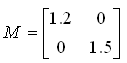Followings are each component matrix of the SVD decomposition calculated with Matlab. Look at each matrix and see if you can visualize the properties of each matrix in your brain. For this reason (for visualizing the matrix in your brain without much calculation), I picked simple 2 x 2 matrix (If you need some practice, refer to Matrix : Transformation page).

 U S V 0     1      1     0 1.5000         0          0    1.2000 0     1      1     0

Following is the result of applying each of these matrix component to an 2 dimensional array of points to help you visualize and understand the meaning of these matrix more intuitively.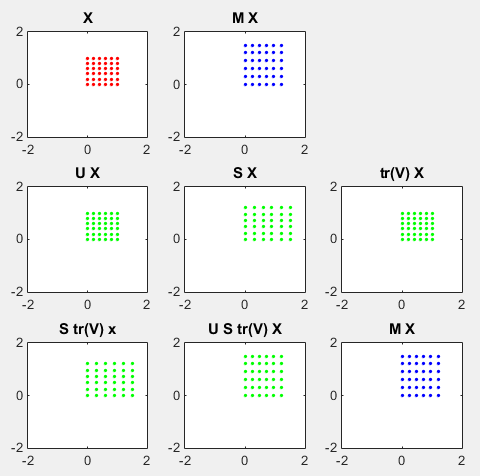Following is another type of presentation. See if you can correlate this with the one above.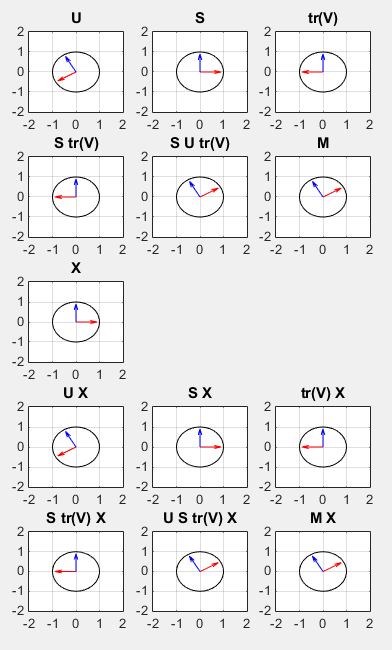Try interpret the following example as described in above example.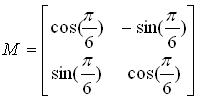Followings are each component matrix of the SVD decomposition calculated with Matlab. Look at each matrix and see if you can visualize the properties of each matrix in your brain. For this reason (for visualizing the matrix in your brain without much calculation), I picked simple 2 x 2 matrix (If you need some practice, refer to Matrix : Transformation page).

 U S V -0.8660   -0.5000    -0.5000    0.8660 1     0      0     1 -1     0      0     1

Following is the result of applying each of these matrix component to an 2 dimensional array of points to help you visualize and understand the meaning of these matrix more intuitively.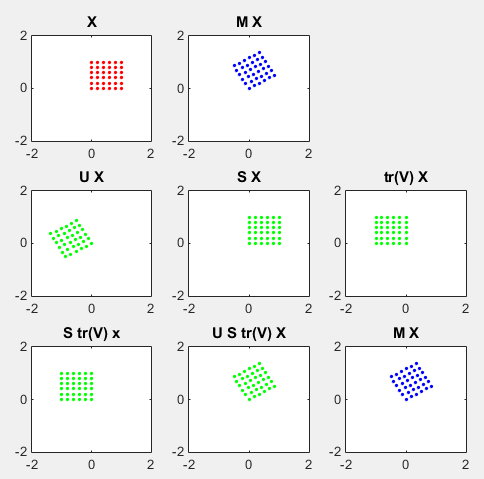Following is another type of presentation. See if you can correlate this with the one above.Try interpret the following example as described in above example.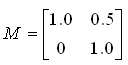Followings are each component matrix of the SVD decomposition calculated with Matlab. Look at each matrix and see if you can visualize the properties of each matrix in your brain. For this reason (for visualizing the matrix in your brain without much calculation), I picked simple 2 x 2 matrix (If you need some practice, refer to Matrix : Transformation page).

 U S V 0.7882   -0.6154     0.6154    0.7882 1.2808         0          0    0.7808 0.6154   -0.7882     0.7882    0.6154

Following is the result of applying each of these matrix component to an 2 dimensional array of points to help you visualize and understand the meaning of these matrix more intuitively.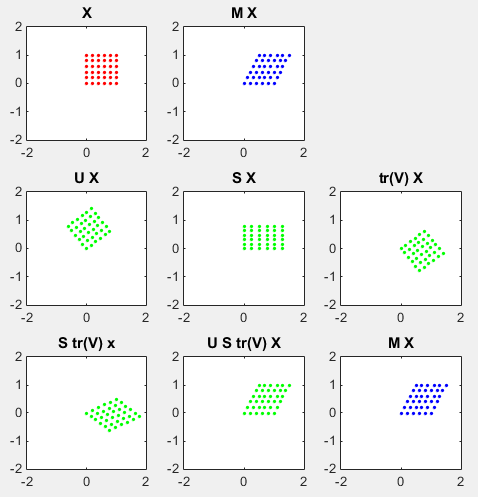Following is another type of presentation. See if you can correlate this with the one above.Applications :

Reference :